# 1.4 Polynomials  (Page 3/15)

 Page 3 / 15

Find the product.

$\left(3x+2\right)\left({x}^{3}-4{x}^{2}+7\right)$

$3{x}^{4}-10{x}^{3}-8{x}^{2}+21x+14$

## Using foil to multiply binomials

A shortcut called FOIL is sometimes used to find the product of two binomials. It is called FOIL because we multiply the f irst terms, the o uter terms, the i nner terms, and then the l ast terms of each binomial.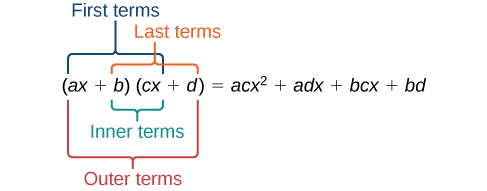The FOIL method arises out of the distributive property. We are simply multiplying each term of the first binomial by each term of the second binomial, and then combining like terms.

Given two binomials, use FOIL to simplify the expression.

1. Multiply the first terms of each binomial.
2. Multiply the outer terms of the binomials.
3. Multiply the inner terms of the binomials.
4. Multiply the last terms of each binomial.
5. Add the products.
6. Combine like terms and simplify.

## Using foil to multiply binomials

Use FOIL to find the product.

$\left(2x-18\right)\left(3x+3\right)$

Find the product of the first terms.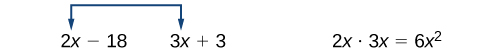Find the product of the outer terms.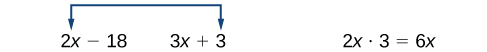Find the product of the inner terms.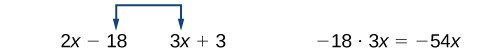Find the product of the last terms.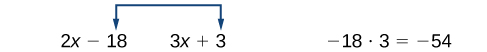Use FOIL to find the product.

$\left(x+7\right)\left(3x-5\right)$

$3{x}^{2}+16x-35$

## Perfect square trinomials

Certain binomial products have special forms. When a binomial is squared, the result is called a perfect square trinomial    . We can find the square by multiplying the binomial by itself. However, there is a special form that each of these perfect square trinomials takes, and memorizing the form makes squaring binomials much easier and faster. Let’s look at a few perfect square trinomials to familiarize ourselves with the form.

Notice that the first term of each trinomial is the square of the first term of the binomial and, similarly, the last term of each trinomial is the square of the last term of the binomial. The middle term is double the product of the two terms. Lastly, we see that the first sign of the trinomial is the same as the sign of the binomial.

## Perfect square trinomials

When a binomial is squared, the result is the first term squared added to double the product of both terms and the last term squared.

${\left(x+a\right)}^{2}=\left(x+a\right)\left(x+a\right)={x}^{2}+2ax+{a}^{2}$

Given a binomial, square it using the formula for perfect square trinomials.

1. Square the first term of the binomial.
2. Square the last term of the binomial.
3. For the middle term of the trinomial, double the product of the two terms.
4. Add and simplify.

## Expanding perfect squares

Expand $\text{\hspace{0.17em}}{\left(3x-8\right)}^{2}.$

Begin by squaring the first term and the last term. For the middle term of the trinomial, double the product of the two terms.

${\left(3x\right)}^{2}-2\left(3x\right)\left(8\right)+{\left(-8\right)}^{2}$

Simplify

$\text{\hspace{0.17em}}9{x}^{2}-48x+64.$

Expand $\text{\hspace{0.17em}}{\left(4x-1\right)}^{2}.$

$16{x}^{2}-8x+1$

## Difference of squares

Another special product is called the difference of squares    , which occurs when we multiply a binomial by another binomial with the same terms but the opposite sign. Let’s see what happens when we multiply $\text{\hspace{0.17em}}\left(x+1\right)\left(x-1\right)\text{\hspace{0.17em}}$ using the FOIL method.

$\begin{array}{ccc}\hfill \left(x+1\right)\left(x-1\right)& =& {x}^{2}-x+x-1\hfill \\ & =& {x}^{2}-1\hfill \end{array}$

The middle term drops out, resulting in a difference of squares. Just as we did with the perfect squares, let’s look at a few examples.

#### Questions & Answers

if 6x=-2 find value of 6/x
issaya Reply
if sin15°=√p, express the following in terms of p
Norman Reply
prove sin²x+cos²x=3+cos4x
Kiddy Reply
the difference between two signed numbers is -8.if the minued is 5,what is the subtrahend
jeramie Reply
the difference between two signed numbers is -8.if the minuend is 5.what is the subtrahend
jeramie
what are odd numbers
micheal Reply
numbers that leave a remainder when divided by 2
Thorben
1,3,5,7,... 99,...867
Thorben
7%2=1, 679%2=1, 866245%2=1
Thorben
the third and the seventh terms of a G.P are 81 and 16, find the first and fifth terms.
Suleiman Reply
if a=3, b =4 and c=5 find the six trigonometric value sin
Martin Reply
ask
Ans
pls how do I factorize x⁴+x³-7x²-x+6=0
Gift Reply
in a function the input value is called
Rimsha Reply
how do I test for values on the number line
Modesta Reply
if a=4 b=4 then a+b=
Rimsha Reply
a+b+2ab
Kin
commulative principle
DIOSDADO
a+b= 4+4=8
Mimi
If a=4 and b=4 then we add the value of a and b i.e a+b=4+4=8.
Tariq
what are examples of natural number
sani Reply
an equation for the line that goes through the point (-1,12) and has a slope of 2,3
Katheryn Reply
3y=-9x+25
Ishaq
show that the set of natural numberdoes not from agroup with addition or multiplication butit forms aseni group with respect toaaddition as well as multiplication
Komal Reply
x^20+x^15+x^10+x^5/x^2+1
Urmila Reply

### Read also:

#### Get Jobilize Job Search Mobile App in your pocket Now!

Source:  OpenStax, Algebra and trigonometry. OpenStax CNX. Nov 14, 2016 Download for free at https://legacy.cnx.org/content/col11758/1.6
Google Play and the Google Play logo are trademarks of Google Inc.

Notification Switch

Would you like to follow the 'Algebra and trigonometry' conversation and receive update notifications?ByByBy OpenStaxByBy DanielrosenbergerBy Rachel CarlisleBy Mackenzie WilcoxBy Danielle StephensBy OpenStaxBy OpenStaxBy Janet ForresterBy OpenStax